# Barometric_Pressure_Sensor_SKU_TOY0058_-DFRobot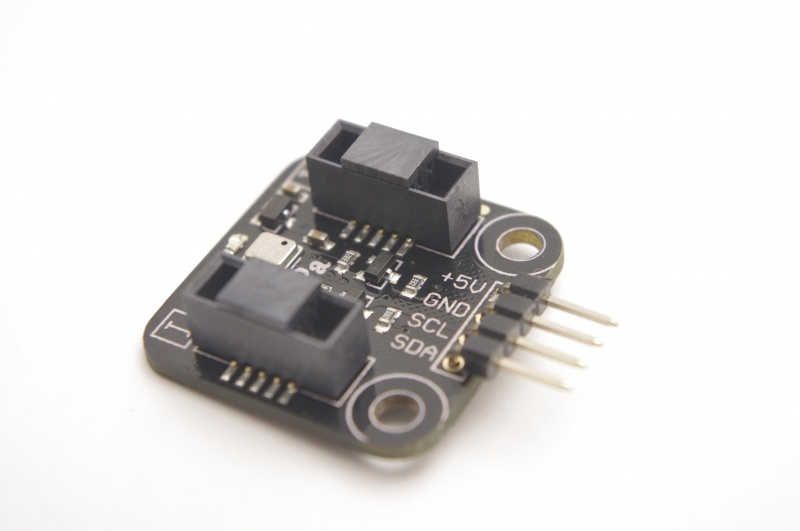## Introduction

Nowadays,there are more and more navigation device with GPS function can be avilabled in electronic market.But along with high precision micro pressure sensor came, a problem solved that we can not know the current altitude,even we can using GPS to determine the location of the equipments.Bosch Sensortec developed a smart, ultra small size, high precision digital sensor BMP180 to fit high precision measurement and data acquisition for intelligence with high accuracy output of pressure (or height) and temperature measurement data.

### Applications

• Temprerature Monitoring
• Pressure Monitoring
• Altitude Monitoring
• 3D navigating in the complex indoor spaces(cooperate with accelerometer)

## Specification

• Input voltage：5V
• Operating temperature：-40～+85 ℃
• measure accuracy：0.12hPa/m
• Interface：I2C Digital Output x1

## Pin Definition

`The definition of Barometric Pressure Sensor sensor pin is`

• 1.+5V
• 2.GND
• 3.SCL
• 4.SDA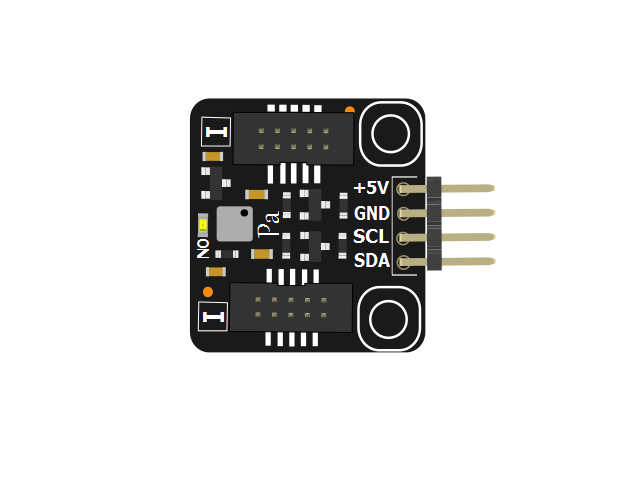## Characteristic Curve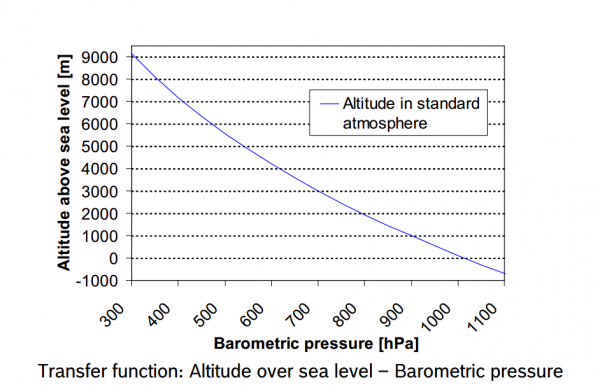## Sample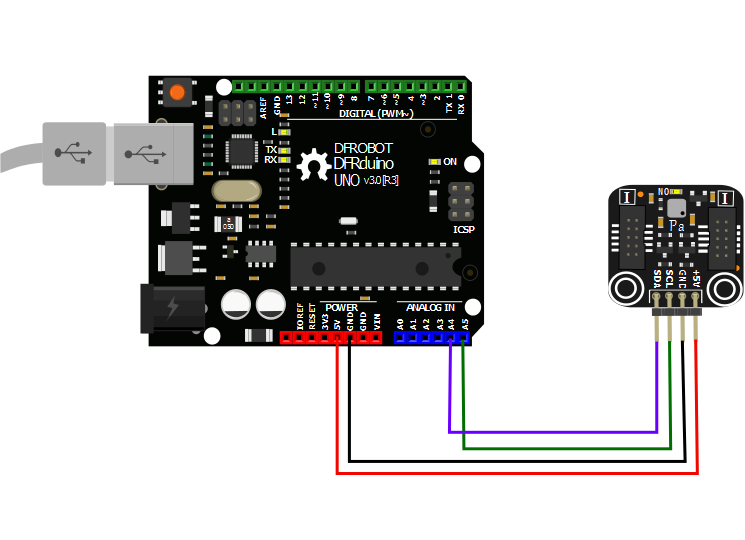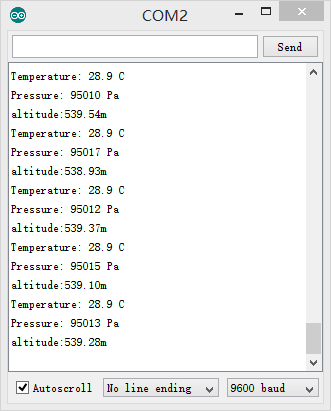### Sample Code

``````/**************************************************************************************/
/**********************DFRobot.com*****************************/
/***write by Tom Riddler          Jun.16.14***/

#include <Wire.h>
//write is (0xEE)     read is (0xEF)
unsigned char OSS;
/**********************MSB      LSB******/
int ac1;           // 0xAA     0xAB
int ac3;           // 0xAE     0xAE
unsigned int ac4;  // 0xB0     0xB1
unsigned int ac5;  // 0xB2     0xB3
unsigned int ac6;  // 0xB4     0xB5
int b1;            // 0xB6     0xB7
int b2;            // 0xB8     0xB9
int mb;            // 0xBA     0xBB
int mc;            // 0xBC     0xBD
int md;            // 0xBE     0xBF
float temperature;
double pressure;
double pressure2;
long b5;
double altitude;

void setup()
{
Serial.begin(9600);
Wire.begin();
OSS = 2;  // Oversampling Setting           0: single    1: 2 times    2: 4 times   3: 8 times
BMP180start();
}

void loop()
{
calculate();
show();
delay(1000);
}

/** calculate centure **/
void calculate()
{
temperature = temperature*0.1;
pressure2 = pressure/101325;
pressure2 = pow(pressure2,0.19029496);
altitude = 44330*(1-pressure2);                            //altitude = 44330*(1-(pressure/101325)^0.19029496);
}

/** print reslut **/
void show()
{
Serial.print("Temperature: ");
Serial.println(" C");
Serial.print("Pressure: ");
Serial.println(" Pa");
Serial.print("altitude:");
Serial.print(altitude);
Serial.println("m");
}

/**BMP180 satrt program**/
void BMP180start()
{                     /*MSB*/
ac1 = bmp180ReadDate(0xAA);                      //get full data
}

/***BMP180 temperature Calculate***/
short bmp180GetTemperature(unsigned int ut)
{
long x1, x2;
x1 = (((long)ut - (long)ac6)*(long)ac5) >> 15;  //x1=((ut-ac6)*ac5)/(2^15)
x2 = ((long)mc << 11)/(x1 + md);                //x2=(mc*2^11)/(x1+md)
b5 = x1 + x2;                                   //b5=x1+x2
return ((b5 + 8)>>4);                           //t=(b5+8)/(2^4)
}

/***BMP180 pressure Calculate***/

long bmp180GetPressure(unsigned long up)
{
long x1, x2, x3, b3, b6, p;
unsigned long b4, b7;

b6 = b5 - 4000;

x1 = (b2 * (b6 * b6)>>12)>>11;
x2 = (ac2 * b6)>>11;
x3 = x1 + x2;
b3 = (((((long)ac1)*4 + x3)<<OSS) + 2)>>2;

x1 = (ac3 * b6)>>13;
x2 = (b1 * ((b6 * b6)>>12))>>16;
x3 = ((x1 + x2) + 2)>>2;
b4 = (ac4 * (unsigned long)(x3 + 32768))>>15;

b7 = ((unsigned long)(up - b3) * (50000>>OSS));
if (b7 < 0x80000000)
p = (b7<<1)/b4;
else
p = (b7/b4)<<1;

x1 = (p>>8) * (p>>8);
x1 = (x1 * 3038)>>16;
x2 = (-7357 * p)>>16;
p += (x1 + x2 + 3791)>>4;

return p;
}

/*** Read 1 bytes from the BMP180  ***/

{
unsigned char data;

Wire.endTransmission();

while(!Wire.available());

}

/*** Read 2 bytes from the BMP180 ***/
{
unsigned char msb, lsb;
Wire.endTransmission();
while(Wire.available()<2);
return (int) msb<<8 | lsb;
}

/*** read uncompensated temperature value ***/
{
unsigned int ut;
Wire.write(0xF4);                       // Write 0x2E into Register 0xF4
Wire.write(0x2E);                       // This requests a temperature reading
Wire.endTransmission();
delay(5);                               // Wait at least 4.5ms
return ut;
}

/*** Read uncompensated pressure value from BMP180 ***/
{
unsigned char msb, lsb, xlsb;
unsigned long up = 0;

Wire.write(0xF4);                        // Write 0x34+(OSS<<6) into register 0xF4
Wire.write(0x34 + (OSS<<6));             // 0x34+oss*64
Wire.endTransmission();
delay(2 + (3<<OSS));                     // Wait for conversion, delay time dependent on OSS

Wire.write(0xF6);                        // Read register 0xF6 (MSB), 0xF7 (LSB), and 0xF8 (XLSB)
Wire.endTransmission();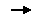Go Shopping Barometric Pressure Sensor(SKU:TOY0058)